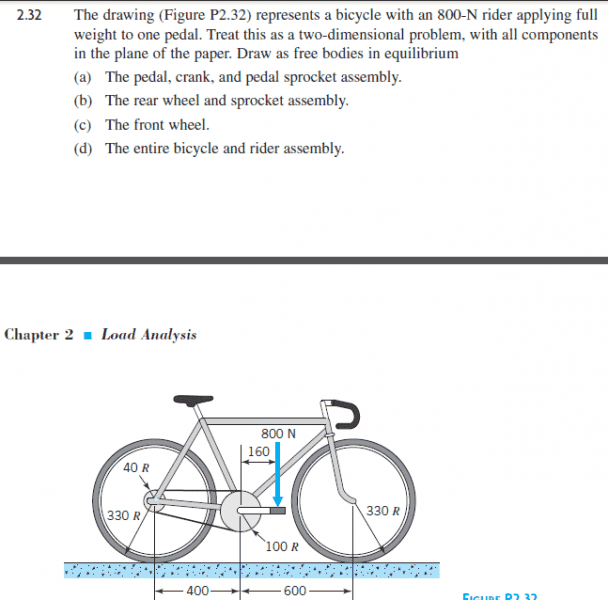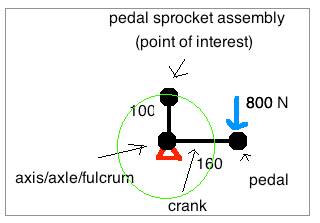# Free Body diagram of bicycle

## Homework Statement

Bicycle with a rider applying weight to on pedal. Draw as free bodies in equilibrium. There are different situations.N/A

## The Attempt at a Solution

So I am working on part a).
1. I believe it is only referring to just the pedal, chain, and the two smaller circles of the (sprocket??).
2. Assuming that is correct to balance the 800N there would need to be an opposing force, is this the torque that is created on the smaller rear circle or is it just as simple as an opposing force in the left side of the pedal?

Doug Huffman
Gold Member
"Pedal, crank and pedal sprocket assembly" aren't standard bicycle terminology. For clarity it should be pedal, crank and chainring. The pedal is where the 800N is applied at the end of the 160mm crank arm opposite its attachment to the 100mm radius chainring. The 800N force is resisted by the resultant tension force in the top/power run of chain.

The smaller circle at the left end of the top chain is the "cog(wheel/set)" where the top chain tension force times the cog radius creates the torque moment on the rear wheel.

The bottom return run of chain is slack.

OmCheeto
Gold Member

## Homework Statement

Bicycle with a rider applying weight to on pedal. Draw as free bodies in equilibrium. There are different situations.

N/A

## The Attempt at a Solution

So I am working on part a).
1. I believe it is only referring to just the pedal, chain, and the two smaller circles of the (sprocket??).
2. Assuming that is correct to balance the 800N there would need to be an opposing force, is this the torque that is created on the smaller rear circle or is it just as simple as an opposing force in the left side of the pedal?

Hello, and welcome to the Forum, ultra york!

Since the system is in equilibrium, all forces and torques must be equal to zero.
So to begin with, you should list, under "Relevant equations", the equation for torque.

It looks to me as though the 800 N force on the pedal is going to create a clockwise torque around the axis of the "pedal sprocket assembly", so you will have to figure out a force at the perimeter of the sprocket that produces a counter-clockwise torque of equal magnitude.

OmCheeto
Gold Member
Since bicycles are a bit weird, I've taken the liberty of drawing the FBD for you.
You can effectively ignore all aspects of the chain, except for the "point of interest".